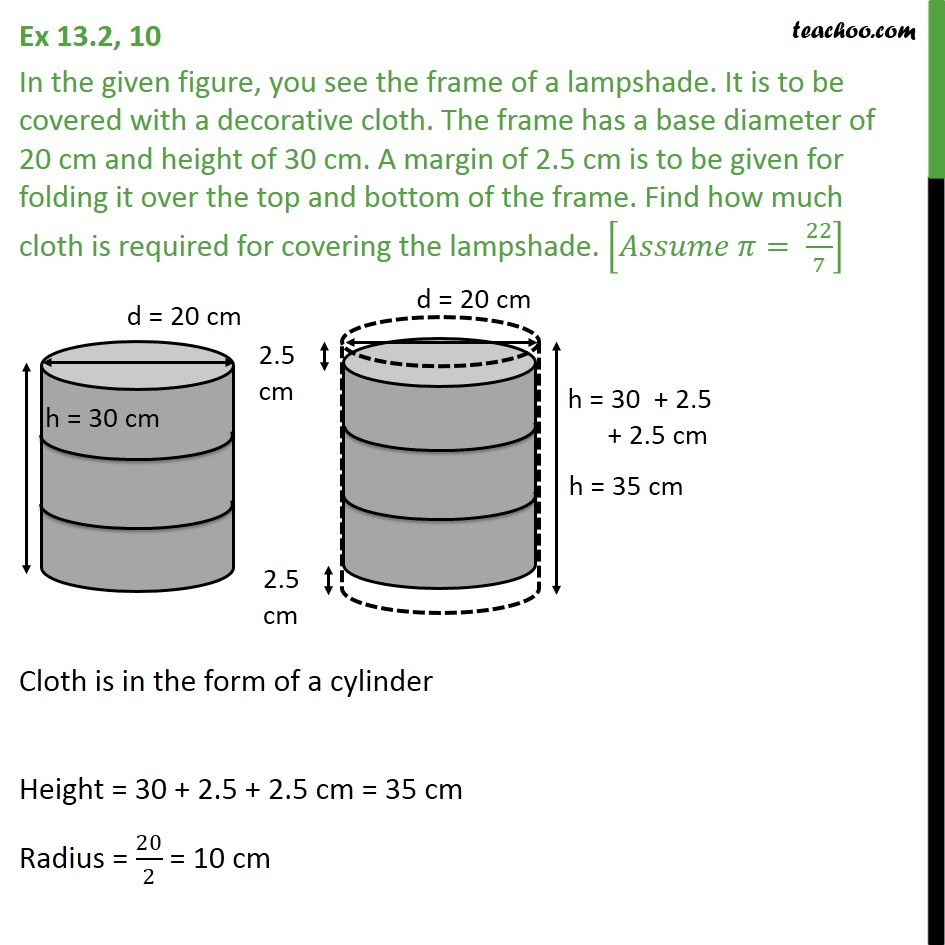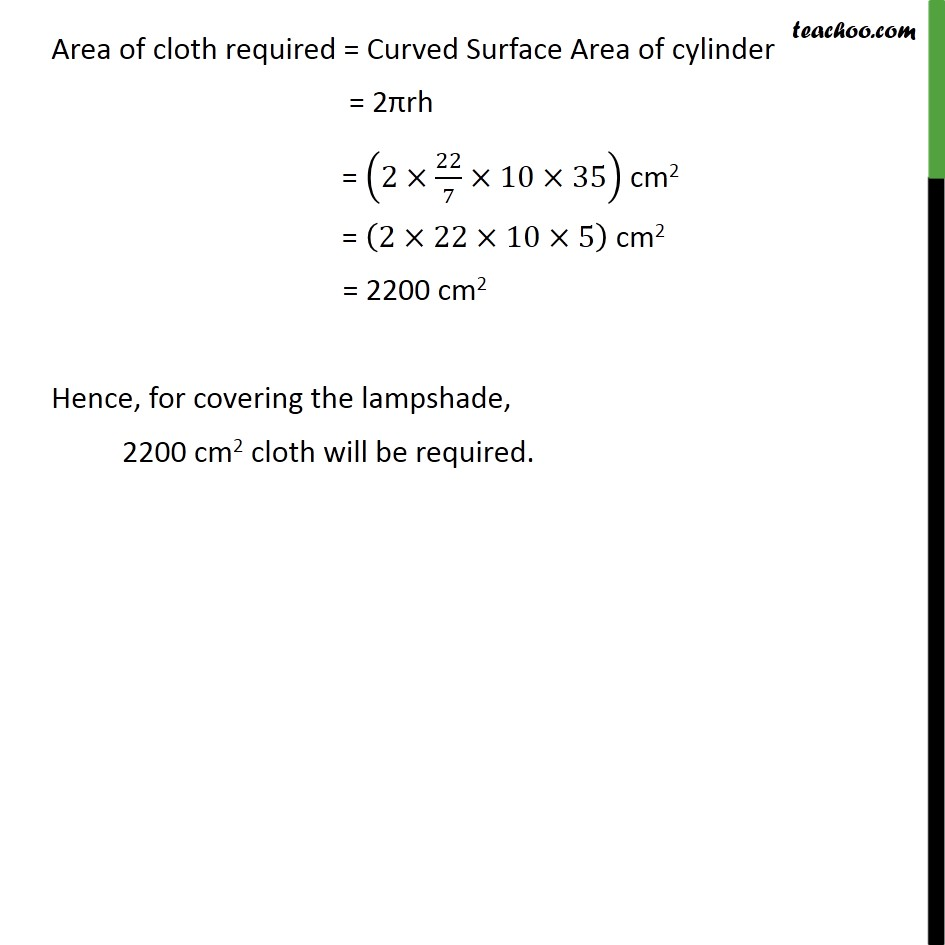1. Chapter 13 Class 9 Surface Areas and Volumes (Term 2)
2. Serial order wise
3. Ex 13.2

Transcript

Ex 13.2, 10 In the given figure, you see the frame of a lampshade. It is to be covered with a decorative cloth. The frame has a base diameter of 20 cm and height of 30 cm. A margin of 2.5 cm is to be given for folding it over the top and bottom of the frame. Find how much cloth is required for covering the lampshade. [ = 22/7] Cloth is in the form of a cylinder Height = 30 + 2.5 + 2.5 cm = 35 cm Radius = 20/2 = 10 cm Area of cloth required = Curved Surface Area of cylinder = 2 rh = (2 22/7 10 35) cm2 = (2 22 10 5) cm2 = 2200 cm2 Hence, for covering the lampshade, 2200 cm2 cloth will be required.

Ex 13.2

Chapter 13 Class 9 Surface Areas and Volumes (Term 2)
Serial order wise